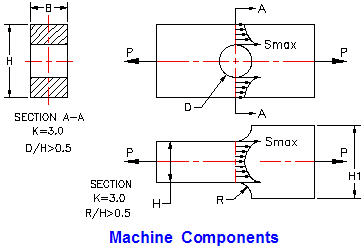Related Resources: material science

### Tension Compression Machine Feature Equation and Calculator

Machine Design and Engineering
Beam Stress Deflection Design and Analysis
Strength of Materials

Design calculators and equations for tension and compression of a machine feature with reduces section area..• Machine components are designed to withstand: applied direct forces, moments and torsion.
• The design load is equal to the applied load multiplied by a combined shock and fatigue factor, Ks.
• The average applied design stress must be multiplied by a stress concentration factor K
• Calculated deflections are compared with required stiffness.
• The material strength is compared with the maximum stress due to combinations of anticipated loads.

As shown below, + P = Tension and - P = Compression

Reference: Design of Machine Elements, by V.M. Faires, published by: The Macmillan Company, New York/Collier-Macmillan Limited, London, England.

All calculators require a Premium Membership